##### Quantum Physics For Dummies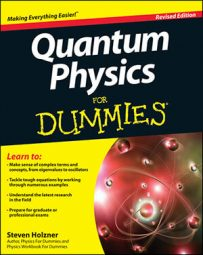When you have the eigenvalues of angular momentum states in quantum mechanics, you can solve the Hamiltonian and get the allowed energy levels of an object with angular momentum. The eigenvalues of the angular momentum are the possible values the angular momentum can take.

Here’s how to derive eigenstate equations with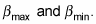Note that L2 – Lz2 = Lx2 + Ly2, which is a positive number, so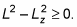That means that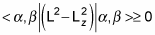And substituting in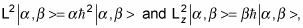and using the fact that the eigenstates are normalized, gives you this: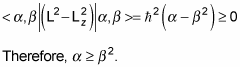So there’s a maximum possible value of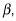which you can call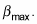You can be clever now, because there has to be a state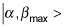such that you can’t raise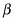any more. Thus, if you apply the raising operator, you get zero: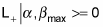Applying the lowering operator to this also gives you zero: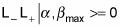And because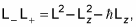that means the following is true: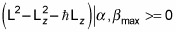Putting in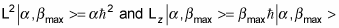gives you this: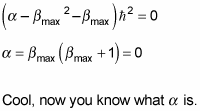At this point, it’s usual to rename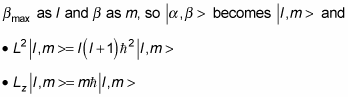You can say even more. In addition to a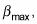there must also be a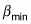such that when you apply the lowering operator, L, you get zero, because you can’t go any lower than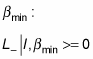And you can apply L+ on this as well: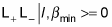From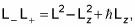you know that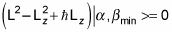which gives you the following: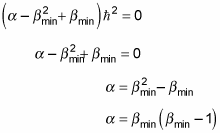And comparing this equation to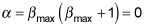gives you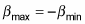Note that because you reach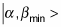by n successive applications of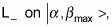you get the following: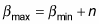Coupling these two equations gives you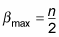Therefore,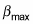can be either an integer or half an integer (depending on whether n is even or odd).

Because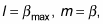and n is a positive number, you can find that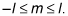So now you have it:

• The eigenstates are | l, m >.

• The quantum number of the total angular momentum is l.

• The quantum number of the angular momentum along the z axis is m.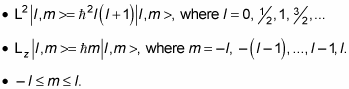For each l, there are 2l + 1 values of m. For example, if l = 2, then m can equal –2, –1, 0, 1, or 2.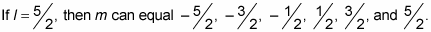You can see a representative L and Lz in the figure.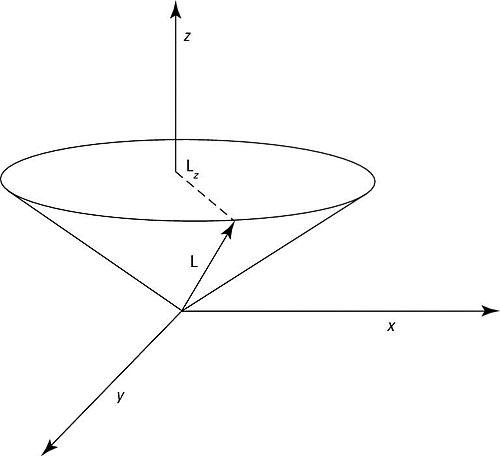L and Lz.

L is the total angular momentum and Lz is the projection of that total angular momentum on the z axis.